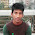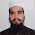### Method and Constructor are working together in c++

Create a suitable situation where Constructor and Method are working together in a class.

Example:

```/* The Circle class (All source codes in one file) (CircleAIO.cpp) */
#include <iostream>    // using IO functions
#include <string>      // using string
using namespace std;

class Circle {
private:
double radius;      // Data member (Variable)
string color;       // Data member (Variable)

public:
// Constructor with default values for data members
Circle(double r = 1.0, string c = "red") {
color = c;
}

double getRadius() {  // Member function/Method
}

string getColor() {   // Member function/Method
return color;
}

double getArea() {    // Member function/Method
}
};   // need to end the class declaration with a semi-colon

// Test driver function or Main Function
int main() {
// Construct a Circle instance
Circle c1(1.2, "blue");
<< " Color=" << c1.getColor() << endl;

// Construct another Circle instance
Circle c2(3.4); // default color
<< " Color=" << c2.getColor() << endl;

// Construct a Circle instance using default no-arg constructor
Circle c3;      // default radius and color
<< " Color=" << c3.getColor() << endl;
return 0;
}```

1.2.ID:201520552
Method and Constructor are working together
https://ideone.com/EHKHRw

3.ID:201610456
creation of situation where method & constructor has been working together:
https://ideone.com/STYOsH

4.ID:201610254

5.6.